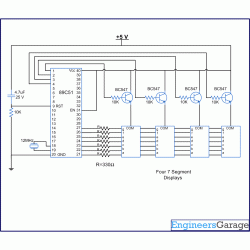# Simple Digital clock using 8051 microcontroller (AT89C51)

Posted by Unknown at 9:34 PM
A digital clock is one that displays time digitally. The circuit explained here displays time with two ‘minutes’ digits and two ‘seconds’ digits on four seven segment displays. The seven segment and switches are interfaced with 8051 microcontroller AT89C51. This circuit can be used in cars, houses, offices etc.

DESCRIBTION:
As soon as the Vcc supply is provided to this circuit, the clock starts from 00:00. The time is displayed on four seven segments (in common anode configuration) by using the concept of multiplexing. This is achieved by using timer interrupt (Timer0) of AT89C51

which is configured to refresh seven segments. The segments are refreshed many times in a second for simultaneous display. The clock runs with a delay of exactly one second. Timer1 has been used to produce a time delay of one second. The data pins (a–h) of all the segments are interconnected and receive signal from port P2 of the microcontroller. The control or enable pins (common anode) are connected to pins 1-4 of port P1 (P1^0 – P1^3).
The number on 4th segment (displaying the unit digit of second) is incremented once in a second as it goes from 0 to 9. The number on 3rd segment is incremented after every 10 seconds from 0 to 5. Thus seconds are displayed varying from 00 to 59. The digit on the 2nd segment changes after every 60 seconds (a minute) from 0 to 9 and so on. Thus the clock runs for an hour and after that it resets to zero againCODE:` `

```// Program to demonstrate the principle of digital clock. this digital clock displays two minute digits and two second digits #include<reg51.h> sbit dig_ctrl_4=P1^0;        //Declaring control pins of the seven segments sbit dig_ctrl_3=P1^1; sbit dig_ctrl_2=P1^2; sbit dig_ctrl_1=P1^3; unsigned char dig_disp=0; int min2; int min1; int sec2; int sec1; char digi_val={0x40,0xF9,0x24,0x30,0x19,0x12,0x02,0xF8,0x00,0x10}; void delay()        //Function to provide a time delay of approx. 1 second. using Timer 1. {     int i;     for(i=0;i<20;i++)     {         TL1=0xFD;          TH1=0x4B;          TR1=1;          while(TF1==0);          TR1=0;          TF1=0;     } } void display() interrupt 1        //Function to display the number using seven segmnet multiplexing. For more details refer seven segment multiplexing. {     TL0=0x36;        //Reloading Timer0     TH0=0xf6;     P2=0xFF;     dig_ctrl_1 = dig_ctrl_3 = dig_ctrl_2 = dig_ctrl_4 = 0;     dig_disp++;     dig_disp=dig_disp%4;     switch(dig_disp)     {         case 0:         P2=digi_val[sec1];         dig_ctrl_1 = 1;         break;            case 1:         P2=    digi_val[sec2];         dig_ctrl_2 = 1;         break;            case 2:         P2=    digi_val[min1];         dig_ctrl_3 = 1;         break;            case 3:         P2=    digi_val[min2];         dig_ctrl_4 = 1;         break;     } } void main() {     TMOD=0x11;        //Intialize Timer 0     TL0=0x36;     TH0=0xF6;     IE=0x82;        // Enable Timer 0 interrupt     TR0=1;        //Start Timer 0     while(1)        //Start clock     {           min2=min1=sec2=sec1=0;           for(min2=0;min2<6;min2++)           {                for(min1=0;min1<10;min1++)                {                 for(sec2=0;sec2<6;sec2++)                 {                      for(sec1=0;sec1<10;sec1++)                      {                         delay();                     }                 }                }           }      } } ```

components:
Transistor BC547
AT89C51 Microcontroller
Seven Segment Display
Tags:

THANKS FOR UR COMMENT ....

## Disclaimer

This blogs is an effort to club the scattered information about engineering and project titles and ideas available in the web. While every effort is made to ensure the accuracy of the information on this site, no liability is accepted for any consequences of using it. Most of the material and information are taken from other blogs and site with the help of search engines. If any posts here are hitting the copyrights of any publishers, kindly mail the details to educations360@gmail.com It will be removed immediately.

## Alexa Rank